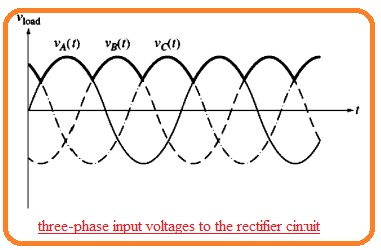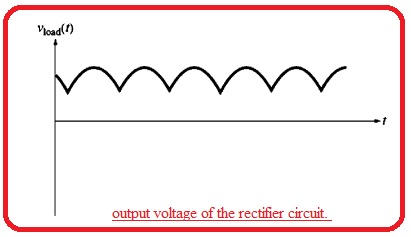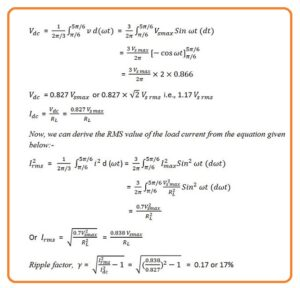Hello, friends welcome to the new post. In this post, we will have a detailed look at Introduction to Three Phase Half Wave Rectifier. The three-phase rectifier is such circuitry that do the rectification of voltage given at ac through three phase transformer and connected with the three diodes that are linked to every 3 phases that exist at the secondary sides or coils. The single-phase rectifier do rectification of ac to dc by the use fo one transformer connected with a single diode at secondary windings.

The disadvantage of this configuration is that has a large value of ripple factors. In this condition half-wave rectify the ripple factors value is 1.21 and for full-wave its value is 0482 For these two rectifiers the impact of ripple factors can not be ignored. But in the case of a half-wave rectifier the value is high of ripple factor but in a full-wave its lesser than a single phase.

## Three Phase Half Wave Rectifier Circuit

• The three-phase half-wave rectifier can be seen here• The factor consisting of 3 diodes having link of cathodes at a single point is that for one point diode having highest voltage given to it will operate and other 2 diodes will be in a reversed biased state
• The three-phase voltages given at the rectifiers circuitry can be seen here,• Its resultant output voltage can here.• Note that voltage at the output of rectifier for any point has a large value than 3 input voltage at that instant.
• The output voltage also has a flat surface than the full-wave bridge rectifier circuit It consists of AC voltage elements at one-eighty hertz and harmonics.
• The ripple factors for rectifier of this category of circuitry is 18.3%
• The value of the three-phase half ave rectifier is determined here and explained with detail.• It can be seen from the above equation that the ripple factor for this rectifier is 0.17.
• In single-phase, rectifier that do half-wave rectification ripple factor is 1.21 and  for sull wave its value is 0.482
• So we can see that the ripple factor in a three-phase rectifier has less value than a single-phase rectifier.
• Further, the frequency of ripples in a three-phase rectifier has a high value. So through that fact these ripples can be easily filtered.
• The ripple frequency in ths state of the three-phase rectifier is 3 times of input frequency.

that is a detailed post about a three-phase half-wave rectifier. If you have any further query ask in the comments. Thanks for reading. Have a nice day.